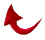IN THE NAME OF GOD MOST GRACIOUS MOST MERCIFUL

JOURNAL OF SUBMISSION

Volume 1 No. 9, November 1993

Editor: Ali R. Fazely, Ph.D.

Associate Editors: Saied Andalib and Milan Sulc

P. O. Box 80333

Baton Rouge, Louisiana 70808

Letter from the Editor.

We humans, as an infinitesimal group of God's creatures, have no choice but absolute submission to God. Our Creator will show us His signs in the horizons and within ourselves until we realize that the Quran is the truth from God . We have witnessed the awesome power and intricacy of the Quran s ULTIMATE MATHEMATICS. This code is based on the PRIME NUMBER 19. This is the foundation upon which this miraculous mathematical code is built. We know that the word "ONE" in Arabic has a value of 19 regardless of numbering the letters of Arabic alphabet sequentially or gematrically. A few years ago the first article about the code of ULTIMATE MATHEMATICS was published in the Submitters' Perspective, entitled "And the Miracle Continues...All Praise Be to God" (see Misc Articles). We have a tendency to think that the miracle is finished.  However, here it comes in all its glory.

I recap for the reader what we already know about "the even and odd". If we look at the number of verses in each sum they are either odd or even. It happens that there are 54 sums which possess an odd number of verses and 60 which possess an even number of verses. If we look at the prime numbers associated with indices 54 and 60, they are: 251 and 281. Now let us add them up:

251 + 281 = 532, and 532 - !9 x 28.

There are 112 verses in the Quran that are not numbered. These are the Basmalah's which are in die beginning of every chapter, except for chapter 9. The Basmalah in chapter 1 is numbered. If we include these Basmalah's in our verse count, we get 52 even and 62 odd number of verses. The 52nd prime is 239 and the 62nd prime is 293. Now let us add them up"

239 + 293 = 532, and 532 = 19 x 28.

We have two pairs of primes, (251,281) and (239,293). If we add the digits of these two pairs of prime numbers we get:

2+5+ 1 +2+8+ 1- 19

and;

2 + 3+ 9+ 2 + 9+ 3-28

Again we are back to 19 and 28. God be praised.

God proves. that He is Omniscient

This is what we knew about the even and the odd (see “The EVEN and the ODD” in the Misc Articles). However, God's system is absolutely beautiful. If we look at the 251st twin prime's companion (TPC) , we find that it is 13398. The 281st TPC is 15732. The 239th TPC is 12162 and the 293rd TPC is 16650. Now we have two pairs of TPC's, (13398, 15732) and (12162, 16650). If we add the digits of these two pairs up, we get:

1 + 3 + 3 + 9 + 8 + 1 + 5 + 7 + 3 + 2 = 42

1 + 2 + 1 + 6 + 2 + 1 + 6 + 6 + 5 + 0 = 30

30 is the 19th composite and 42 is the 28th composite. God be glorified. There are no known mathematical relations connecting these numbers. Therefore, "we have to submit to God ....

I thought I was finished, but truly God is omniscient. The 54th TPC is 1698, and the 60th TPC is 1950. The 52nd TPC is 1620 and the 62nd TPC is 2028. We have two pairs of TPC's namely, (1698, 1950) and (1620, 2028). Now let us add these numbers up, we get"

1698 + 1950  = 3648 = 192 x 19

1620 + 2028  = 3648 = 192 x 19

Note that 192 is a TPC and its index is 14. We know that 19 is the 8th prime number. Furthermore, 148 is the index of composite 192. Praise be to God.

Last month Saied Andalib noted that in sura 57 iron is mentioned in verse 25 and that the last word God mentioned in verse 25 is the 26th word God from the beginning of this sura. This is the number of protons in the iron nucleus. He also noted that verse 25 is the 19th verse which contains the word God in this sura. God is omniscient.

Chapter 36 called Y.S. is the 19th initialed chapter in the Quran. The number of initials are 285 which is 19 x 15. But why 15 as the coefficient of 19? We know that 19 is the 8th prime number. 15 happens to be the 8th composite. We also know that 36 is the sum of all integers up to and including 8. This means that 1 + 2 + 3 + 4 + 5 + 6 + 7 + 8 = 36 . If we concatenate 19 and 8 we get 198. The 152nd composite is 198 and 152 = 19 x 8. Milan noted that if we concatenate 15 and 8, we get 158 which is the 120th composite and 120 = 15 x 8. The uniqueness of this mathematics is truly mind boggling. Let us praise God.

I include a copy of the paper (see, “A Relation Between Twin Primes and their Indices” in the Misc Articles section), that I am trying to publish in a refereed journal: The mathematics you are just about to witness is unique since Gauss's "Prime Number Theorem". This is new mathematics and we are blessed to see it in this issue of Journal of Submission. All praises and thanks are due to God, Creator of all things.

.

References

 Quran, 41:53

 Journal of Submission, Vol. 1, No. 5, July (1993)

 Journal of Submission, Vol. 1, No. 4, June (1993)Return to Table of Contents